# House Prices: Advanced Regression Techniques

### Goal¶

The dataset contains 79 explanatory variables that describe alsmost every aspect of residential homes in Ames, Iowa. It's up to me to predict the pricing of houses based on the given dataset. The data is split into a train and test data set for me to train and test on. This dataset is part of a kaggle competition in which the principles of stacking, blending and ensembling techniques can be learned. Furthermore, for this dataset (as you will see) feature engineering will be crucial to getting a better RMSE.

Main problem of dataset:
There seems to be some underlying problem with this dataset: missing values. The amount of training data is very small, so filling in the missing data is important to increase the accuracy of the model. Thus, it may be that feature engineering/feature selection and missing data imputation are more important than modelling.

I will start by loading some basic packages that will be used for most operations. It should be noted that you have to have Xgboost installed for this notebook to work, which you can do below. Moreover, getting rpy2 installed might prove to be tricky. The plots can also be done in seaborn if neccesary.

In :
try:
import pandas as pd
import numpy as np
import seaborn as sns
import matplotlib.pyplot as plt
from scipy.stats import skew
from sklearn.model_selection import cross_val_score

%matplotlib inline
except:
import pip
pip.main(['install', 'seaborn'])
pip.main(['install', 'xgboost'])
pip.main(['install', 'rpy2'])

import pandas as pd
import numpy as np
import seaborn as sns
import matplotlib.pyplot as plt
from scipy.stats import skew
from sklearn.model_selection import cross_val_score

%matplotlib inline

The rpy2.ipython extension is already loaded. To reload it, use:


The data (as previously mentioned) is split into a train and test file with both the features and target variable available in the train.csv file to train on and only the features (without the target variable) in the test set. Although I have loaded the files, it is still necessary to also load them in R, which takes a few seconds.
The data can be found here: https://www.kaggle.com/c/house-prices-advanced-regression-techniques

In :
train = pd.read_csv('train.csv')

In :
%%R -i train -i test
library(ggplot2)


### Visualization¶

The first part of this analyis is to explore and visualize the data. I will use both seaborn and ggplot2 since one may be preferred over the other in some cases. There are many features to explore and I will try to only keep the focus on those that have some interesting relationship with the target variable "SalePrice". Furthermore, the focus will be on trying to understand the distribution and quality of the data so that I can improve the modeling process.

I will start with a basic exploration of the target variable "SalePrice" and plot its distribution to gain some information.

In :
%%R -w 1000 -h 450
ggplot(train, aes(x=SalePrice)) +
geom_histogram(color = 'black', fill = 'grey', bins = 50) +
theme_bw() +
labs(x = 'Sale Price', title ="Sales Price Distribution") +
theme(legend.position="none", axis.text=element_text(size=14), axis.title=element_text(size=16,face="bold"),
plot.title = element_text(lineheight=1, face="bold", size=20, hjust = 0.5))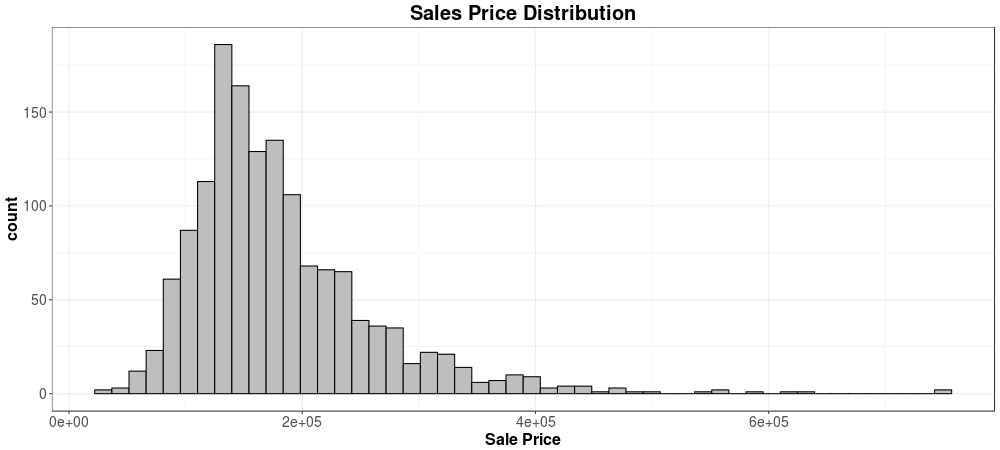You can see that the target variable "SalePrice" has a skewed distribution. Because I will be predicting a continuous feature, I will be using regressors which perform better if the target variable has a normal distribution with little to no skewdness. Thus, it is important to remember that in a later stage the data needs to be normalized.

### Numeric Features¶

Next, I will focus on the numeric features and see in what way they are related to the target variable. To do this, a correlation matrix is often the first step just to see what is exactly going on with the data. After that, I will look into some individual plots showing more on the relationship between that feature and the target.

In :
correlation_matrix = train.corr()
f, axis = plt.subplots(figsize=(8, 6))
sns.heatmap(correlation_matrix, vmax=.6, square=True);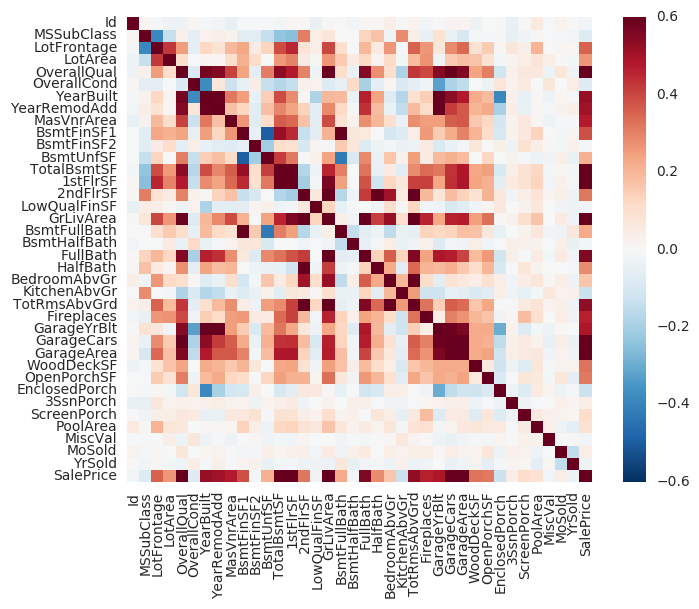There's a lot going on the in the correlation matrix. We see that there are some clusters that are highly correlated like GarageArea and GarageCars, which makes sense since having a garage that can hold more cars would likely also have a greater area. It is possible that there's some multicollinearity which affects the results.

Next, let's take a look at the features that are highly correlated with each other and look at those in more detail. I will select features that have a pearson's correlation of .7 or more.

In :
# Show highest correlation in the correlation matrix
correlation_matrix = train.corr().abs()
unstacked = correlation_matrix.unstack()
sort = unstacked.sort_values(kind="quicksort")
sort[(sort < 1) & (sort > 0.7)]

Out:
SalePrice     GrLivArea       0.708624
GrLivArea     SalePrice       0.708624
SalePrice     OverallQual     0.790982
OverallQual   SalePrice       0.790982
TotalBsmtSF   1stFlrSF        0.819530
1stFlrSF      TotalBsmtSF     0.819530
TotRmsAbvGrd  GrLivArea       0.825489
GrLivArea     TotRmsAbvGrd    0.825489
YearBuilt     GarageYrBlt     0.825667
GarageYrBlt   YearBuilt       0.825667
GarageArea    GarageCars      0.882475
GarageCars    GarageArea      0.882475
dtype: float64

Some things seems to be going on here:

• There is some multicollinearity within the "Garage" features.
• SalePrice is higly correlated with OverallQual and GrLivArea
• Some people have bought a house without a garage and built the garage in later, which is why the correlation isn't 1

Next, I will look at all the features that have a high correlation with SalePrice.

In :
sort['SalePrice'][sort['SalePrice']>0.6]

Out:
1stFlrSF       0.605852
TotalBsmtSF    0.613581
GarageArea     0.623431
GarageCars     0.640409
GrLivArea      0.708624
OverallQual    0.790982
SalePrice      1.000000
dtype: float64

The overall quality of the house has the highest correlation with the sale price. The Garage seems to be an important feature, as well as the number of floors and whether it has a basement or not. Although we have a lot of numbers telling us a lot of things, it would be more intuitive if the data was actually visualized. The type of relation is not clear purely based on pearson's r, thus I will use a pairplot to plot all features that are highly correlated with SalePrice.

In :
sns.set_style("whitegrid")
cols = sort['SalePrice'][sort['SalePrice']>0.6].index
sns.pairplot(train[cols], size = 2.5)
plt.show()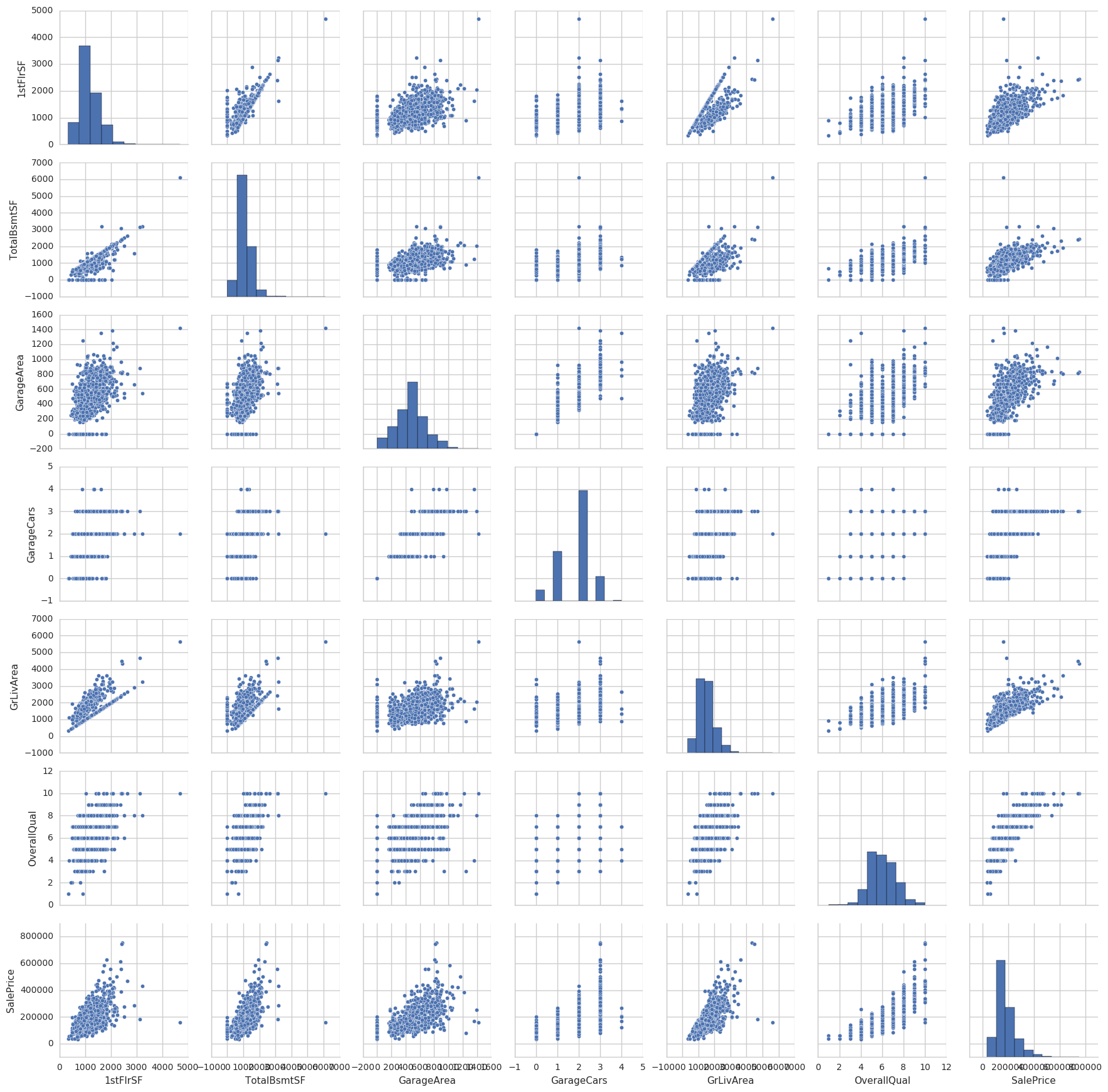Although we now can clearly see the relationships, I also notice that a lot of features have some heteroscedasticity and some are not normally distributed. It might be worth to look into those features in more detail.

In :
%%R -w 700 -h 450
ggplot(train, aes(x=YearBuilt, y=GarageYrBlt)) +
geom_point() +
theme_bw() +
labs( y = "Year built (garage)", x = 'Year built (house)', title ="Year built of garage versus house") +
theme(legend.position="none", axis.text=element_text(size=14), axis.title=element_text(size=16,face="bold"),
plot.title = element_text(lineheight=1, face="bold", size=20, hjust = 0.5))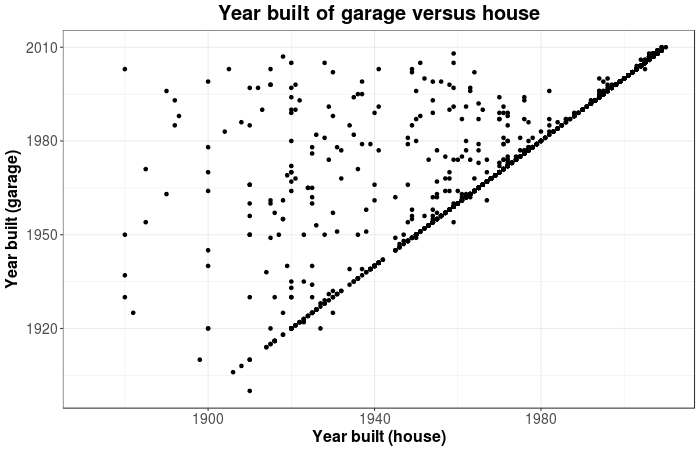This is really interesting to see! What happened is that most garages are built the same year as the house. However, some people either extended their garage or didn't have one and built that later. Everything on the line are the houses that had a garage from the beginning and most other point are those garages that were built later than the house.

In :
%%R -w 700 -h 450
ggplot(train, aes(y=SalePrice, x=GrLivArea)) +
geom_point() +
theme_bw() +
labs( y = "Sales price", x = 'Living Area', title ="Sales price per living area") +
theme(legend.position="none", axis.text=element_text(size=14), axis.title=element_text(size=16,face="bold"),
plot.title = element_text(lineheight=1, face="bold", size=20, hjust = 0.5))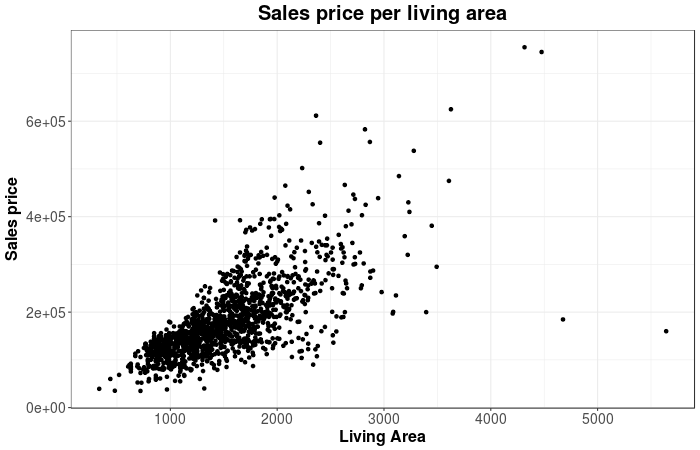There are two things that you should see immediately:

• There are two outliers on the far right (which we will deal with at a later stage)
• There is some heteroscedasticity going on (which we will also deal with later)
In :
%%R -w 700 -h 450
ggplot(train, aes(x=SalePrice, y=OverallQual)) +
geom_count() +
theme_bw() +
labs( x = "Sales price", y = 'Overal Quality', title ="Sales price on overall quality") +
theme(legend.position="none", axis.text=element_text(size=14), axis.title=element_text(size=16,face="bold"),
plot.title = element_text(lineheight=1, face="bold", size=20, hjust = 0.5))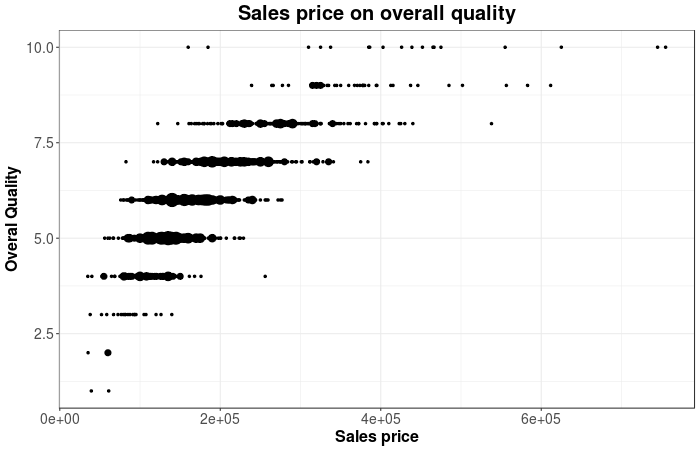There clearly seems to be some relationship between overal quality and the sales price of the house such that an increase in sales price suggest an increase in overal quality.

### Categorical Features¶

Categorical features have been missing from the visualisation so far. Since there are too many combinations of features to visualize, I will focus on the categorical features that may (according to my intuition) be related to SalesPrice. Below is a list of categorical features in the dataset.

In :
train.dtypes[train.dtypes == "object"]

Out:
MSZoning         object
Street           object
Alley            object
LotShape         object
LandContour      object
Utilities        object
LotConfig        object
LandSlope        object
Neighborhood     object
Condition1       object
Condition2       object
BldgType         object
HouseStyle       object
RoofStyle        object
RoofMatl         object
Exterior1st      object
Exterior2nd      object
MasVnrType       object
ExterQual        object
ExterCond        object
Foundation       object
BsmtQual         object
BsmtCond         object
BsmtExposure     object
BsmtFinType1     object
BsmtFinType2     object
Heating          object
HeatingQC        object
CentralAir       object
Electrical       object
KitchenQual      object
Functional       object
FireplaceQu      object
GarageType       object
GarageFinish     object
GarageQual       object
GarageCond       object
PavedDrive       object
PoolQC           object
Fence            object
MiscFeature      object
SaleType         object
SaleCondition    object
dtype: object

I will take a look at the following categories: SaleCondition and HouseStyle. Furthermore, I will see how they influence the visualizations that we've seen starting with SalePrice, LivingArea and SaleCondition.

In :
%%R -w 1000 -h 600
ggplot(train, aes(y=SalePrice, x=GrLivArea, color = factor(SaleCondition))) +
geom_point() +
theme_bw() +
labs( x = "Living Area" , y = 'Sale Price', title ="Living Area on Sale Price by Condition") +
theme(axis.text=element_text(size=14), axis.title=element_text(size=16,face="bold"),
plot.title = element_text(lineheight=1, face="bold", size=20, hjust = 0.5))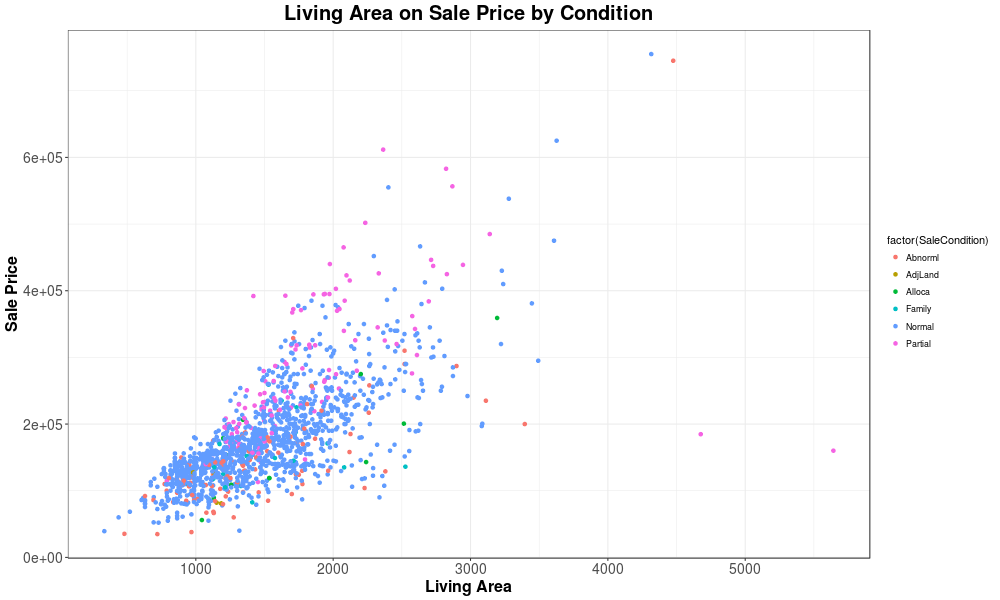The graph doesn't give much information. It may be then that the salecondition doens't influence the relationship between living area and saleprice. The main conclusion is that most houses seem to be of condition "Normal". Perhaps we see something different for neighborhood.

In :
%%R -w 1000 -h 600
ggplot(train, aes(y=SalePrice, x=GrLivArea, color = factor(HouseStyle))) +
geom_point() +
theme_bw() +
labs( x = "Living Area" , y = 'Sale Price', title ="Living Area on Sale Price by House Style") +
theme(axis.text=element_text(size=14), axis.title=element_text(size=16,face="bold"),
plot.title = element_text(lineheight=1, face="bold", size=20, hjust = 0.5))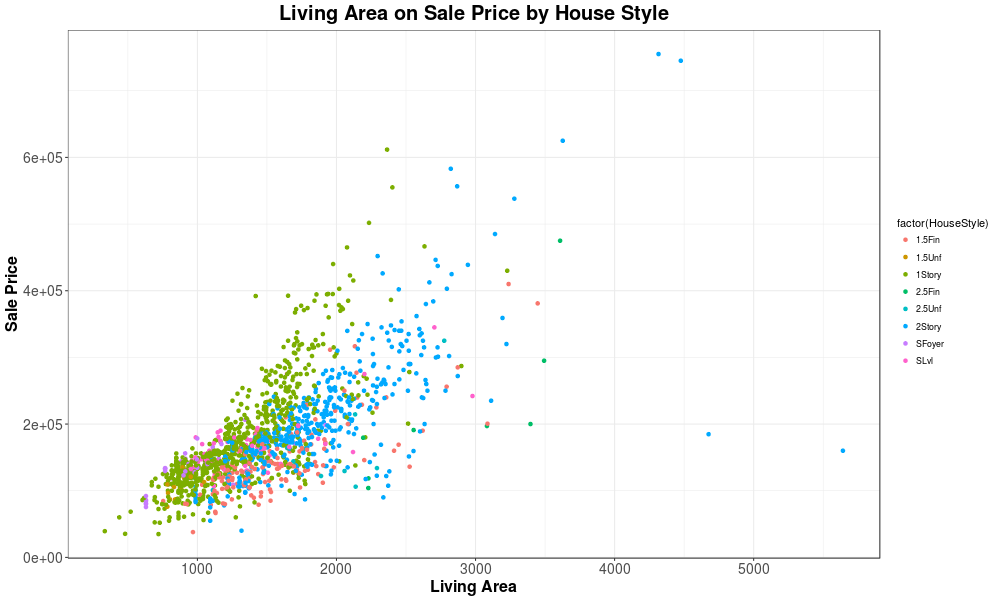There's definitely something different going on here. The 1 story houses are according to the plot the most expensive houses for each square feet of living area. You would get more area per dollar if you would buy a 2 story house.

# Feature Engineering¶

Now that I've finished exploring the data it is time to work on some feature engineering. During the exploration several things came to my attention that I need to work on:

• There were some outliers
• The target feature is not normally distributed
• Some features showed heteroscedasticity
• Multicollinearity
• Although multicollinearity was found in some features, I did not find any improvement in the scores if I deleted those features. Therefore, I simply let them be.

Furthermore, I will be looking at:

• Variance of features
• Missing Values

### Outliers¶

In this machine learning task, it will be likely that I'll be using some form of regression analysis. These kind of methods are very sensitive to outliers, which may decrease the models accuracy. Therefore, I'll be looking for any outliers and remove them if neccesary.

In :
%%R -w 700 -h 450
ggplot(train, aes(y=SalePrice, x=GrLivArea)) +
geom_point() +
theme_bw() +
labs( y = "Sales price", x = 'Living Area', title ="Sales price per living area") +
theme(legend.position="none", axis.text=element_text(size=14), axis.title=element_text(size=16,face="bold"),
plot.title = element_text(lineheight=1, face="bold", size=20, hjust = 0.5))There seems to be 2 extreme outliers on the bottom right, really large houses that sold for really cheap. Seeing the trends in the plot above I believe these two points to be outliers and not representative for the entire dataset. Thus, my initial conclusion is to delete those outliers. Luckily, the author of the dataset recommends removing 'any houses with more than 4000 square feet' from the dataset which confirms my reasoning.
Reference : https://ww2.amstat.org/publications/jse/v19n3/decock.pdf

In :
train.drop(train[train["GrLivArea"] > 4000].index, inplace=True)

In :
%%R -w 700 -h 450 -i train -i test
ggplot(train, aes(y=SalePrice, x=GrLivArea)) +
geom_point() +
theme_bw() +
labs( y = "Sales price", x = 'Living Area', title ="Sales price per living area") +
theme(legend.position="none", axis.text=element_text(size=14), axis.title=element_text(size=16,face="bold"),
plot.title = element_text(lineheight=1, face="bold", size=20, hjust = 0.5))